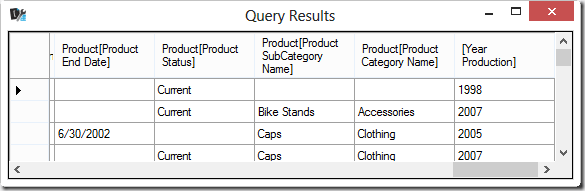UPDATE 2016-07-23 : Please note certain syntaxes changed behavior in recent builds of SSAS Tabular 2012/2014 and in SSAS 2016. See other notes later in this article.

UPDATE 2017-01-30 : Excel 2016, Power BI and SSAS Tabular 2016 now have SUMMARIZECOLUMNS, which should replace the use of ADDCOLUMNS/SUMMARIZE described in this article. Read more in Introducing SUMMARIZECOLUMNS.

NOTE: all the queries included in this article can be tried querying the AdventureWorks Tabular Model you can download from Codeplex. All the outputs are produced by using DaxStudio, our favorite free DAX editor.

## Extension Columns

Extension columns are columns that you add to existing tables. You can obtain extension columns by using both ADDCOLUMNS and SUMMARIZE. For example, the following query adds a Year Production column to the rows returned from the Product table.

```EVALUATE
Product,
"Year Production", YEAR( Product[Product Start Date] )
)```You can also create an extension column by using SUMMARIZE. For example, you can count the number of products for each product category by using the following query (please note that this query is not a best practice – you will see why later in this article).

```EVALUATE
SUMMARIZE(
Product,
Product[Product Category Name],
"Products", COUNTROWS( Product )
)
```In practice, an extension column is a calculated column created within the query.

## Query Projection

In a SELECT statement in SQL, you can choose the column projected in the result, whereas in DAX you can only add columns to a table by creating extension columns. The only workaround available is to use SUMMARIZE to group the table by the columns you want to obtain in the output. As long as you do not need to see duplicated rows in the result, this solution does not have particular side effects. For example, if you want to get just the list of product names and their corresponding production start date, you can write the following query.

```EVALUATE
SUMMARIZE(
Product,
Product[Product Name],
Product[Product Start Date]
)
```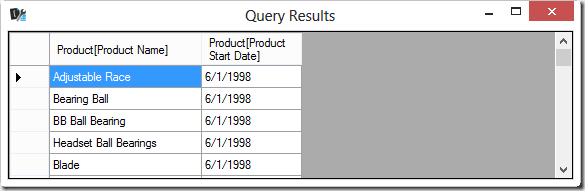Whenever you can create an extended column by using both ADDCOLUMNS and SUMMARIZE, you should always favor ADDCOLUMNS for performance reasons. For example, you can add the year of production start date by using one of two techniques. First, you can just use SUMMARIZE.

```EVALUATE
SUMMARIZE(
Product,
Product[Product Name],
Product[Product Start Date],
"Year Production", YEAR( Product[Product Start Date] )
)
```

Second, you can use ADDCOLUMNS adding the Year Production column to the SUMMARIZE result.

```EVALUATE
SUMMARIZE(
Product,
Product[Product Name],
Product[Product Start Date]
),
"Year Production", YEAR( Product[Product Start Date] )
)
```

Both queries produce the same result.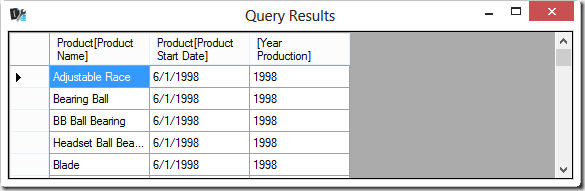However, you should always favor the ADDCOLUMNS version. The rule of thumb is that you should never add extended columns by using SUMMARIZE, unless it is required due to at least one of the following conditions:

• You want to use ROLLUP over one or more grouping columns in order to obtain subtotals
• You are using non-trivial table expressions in the extended column, as you will see in the “Filter Context in SUMMARIZE and ADDCOLUMNS” section later in this article

The best practice is that, whenever possible, instead of writing

`SUMMARIZE( <table>, <group_by_column>, <column_name>, <expression> )`

you should write:

```ADDCOLUMNS(
SUMMARIZE( <table>, <group by column> ),
<column_name>, CALCULATE( <expression> )
)
```

The CALCULATE you can see in the best practices template above is not always required, but you need it whenever the <expression> contains an aggregation function. The reason is that ADDCOLUMNS operates in a row context that does not automatically propagate into a filter context, whereas the same <expression> within a SUMMARIZE is executed into a filter context corresponding to the values in the grouped columns. The previous examples used a scalar expression over a column that was included in the SUMMARIZE output, so the reference to the column value was valid within the row context. Now, consider the following query that you have already seen at the beginning of this article.

```EVALUATE
SUMMARIZE(
Product,
Product[Product Category Name],
"Products", COUNTROWS( Product )
)
```

If you rewrite this query by simply moving the Products extended columns out of the SUMMARIZE into an ADDCOLUMNS function, you obtain the following query that produces the wrong result. This is because it returns the number of rows in the entire Products table for each row of the result instead of returning the number of products for each category.

```EVALUATE
SUMMARIZE(
Product,
Product[Product Category Name]
),
"Products", COUNTROWS( Product )
)
```In order to obtain the result you want, you have to wrap the expression for the Products extended column within a CALCULATE statement. This way, the row context for Product Category Name is transformed into a filter context and the COUNTROWS function only considers the products belonging to the category of the current row.

```EVALUATE
SUMMARIZE(
Product,
Product[Product Category Name]
),
"Products", CALCULATE( COUNTROWS( Product ) )
)
```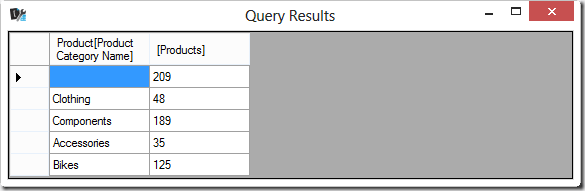Thus, as a rule of thumb, wrap any expression for an extended column within a CALCULATE function whenever you move an extended column out from SUMMARIZE into an ADDCOLUMN statement.

## Grouping by Extension Columns

UPDATE 2016-07-23 : Recent versions of SSAS Tabular 2012/2014 and SSAS Tabular 2016 aggregate correctly by using extension columns. Please try the following code with your build of SSAS Tabular and carefully consider data lineage in SSAS Tabular 2016 for similar issues.

A counterintuitive limitation in DAX is that you can group by extension columns, but you cannot perform meaningful calculations grouping by extension columns. For example, consider an extended column added to the Internet Sales table that returns the range of unit prices obtained with a logarithmic expression. In practice, any sale made with a unit price between 0 and 1 will be grouped as 1, between 1 and 10 will be grouped as 10, between 10 and 100 will be grouped as 100, and so on.

```EVALUATE
'Internet Sales',
"Price Level", POWER( 10, 1 + INT( LOG10( 'Internet Sales'[Unit Price] ) ) )
)
```

You can group data by using the Price Level extension column in a SUMMARIZE expression, so that you can see what the groups are for existing sales.

```EVALUATE
SUMMARIZE(
'Internet Sales',
"Price Level", POWER( 10, 1 + INT( LOG10( 'Internet Sales'[Unit Price] ) ) )
),
[Price Level]
)
ORDER BY [Price Level]
```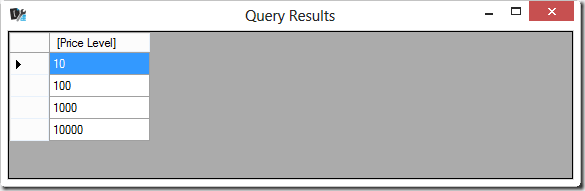However, the extended columns that you can use in a SUMMARIZE expression are not part of the filter context. Thus, if you try to add an extended column to a SUMMARIZE expression that groups by Price Level, the expression cannot be grouped by Price Level and produces an unexpected result.

```EVALUATE
SUMMARIZE(
'Internet Sales',
"Price Level", POWER( 10, 1+INT( LOG10( 'Internet Sales'[Unit Price] ) ) )
),
[Price Level],
"Total Sales", SUM( 'Internet Sales'[Sales Amount] )
)
ORDER BY [Price Level]
```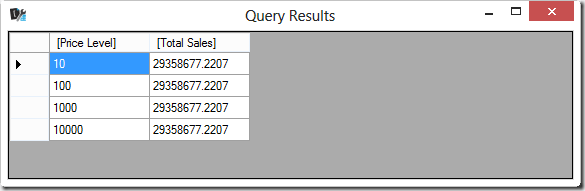The Total Sales extended column always contains the sum of Sales Amount for all the rows of the Internet Sales table, regardless of the Price Level. This is completely counterintuitive. Indeed, you can see different lines but it is as though the Price Level column does not belong to the Internet Sales table and is instead in a separate table unrelated to Internet Sales – so that its filter context does not propagate to Internet Sales.

Note: in future versions of Analysis Services, the query you have just seen might produce warnings or errors instead of returning this unexpected result.

For this reason, trying to use CALCULATE and ADDCOLUMNS such as in the following query produces the same result as the previous query, which is not what we would like to see.

```EVALUATE
SUMMARIZE(
'Internet Sales',
"Price Level", POWER( 10, 1 + INT( LOG10( 'Internet Sales'[Unit Price] ) ) )
),
[Price Level]
),
"Total Sales", CALCULATE( SUM( 'Internet Sales'[Sales Amount] ) )
)
ORDER BY [Price Level]
```

Since you do not have a relationship between two tables – Internet Sales and the “virtual” one for Price Level – you have to inject a filter condition within the CALCULATE expression. You would do this in order to only consider the rows in Internet Sales whose price is included within the level defined by Price Level. A simple way to do that is repeating the expression that calculates the Price Level in the filter expression, as in the following query.

```EVALUATE
SUMMARIZE(
'Internet Sales',
"Price Level", POWER( 10, 1 + INT( LOG10( 'Internet Sales'[Unit Price] ) ) )
),
[Price Level]
),
"Total Sales",
CALCULATE(
SUM( 'Internet Sales'[Sales Amount] ),
FILTER(
'Internet Sales',
[Price Level]
= POWER( 10, 1 + INT( LOG10( 'Internet Sales'[Unit Price] ) ) )
)
)
)
ORDER BY [Price Level]
```

In order to avoid the duplication of an expression, you can use the DEFINE MEASURE syntax.

```DEFINE
MEASURE 'Internet Sales'[Price Band]
= POWER( 10, 1 + INT( LOG10( VALUES( 'Internet Sales'[Unit Price] ) ) ) )
EVALUATE
SUMMARIZE(
'Internet Sales',
"Price Level", [Price Band]
),
[Price Level]
),
"Total Sales",
CALCULATE(
SUM( 'Internet Sales'[Sales Amount] ),
FILTER( 'Internet Sales', [Price Level] = [Price Band] )
)
)
ORDER BY [Price Level]
```

Both previous queries return the expected result, showing the sum of Sales Amount for each price level.The main takeaway is that you have to generate the proper filter context in any calculation based on the grouping of an extended column, because it does not affect the filter context of the table it has been added to.

## Measure’s Syntax Observations

You might wonder why we did not use the same Price Level name for both the local measure and the extended column names. The reason is that even if it is possible, it would make the query harder to read. In fact, you can try the previous query using Price Level instead of Price Band to name the local measure, as follows.

```DEFINE
MEASURE 'Internet Sales'[Price Level]
= POWER( 10, 1 + INT( LOG10( VALUES( 'Internet Sales'[Unit Price] ) ) ) )
EVALUATE
SUMMARIZE(
'Internet Sales',
"Price Level", [Price Level]
),
[Price Level]
),
"Total Sales",
CALCULATE(
SUM( 'Internet Sales'[Sales Amount] ),
FILTER( 'Internet Sales', [Price Level] = [Price Level] )
)
)
ORDER BY [Price Level]
```

However, the query written this way does not work, because the highlighted condition contained in the filter statement will always returns true – producing a wrong result.In this case, the EARLIER statement would not help you. The problem is that, as a best practice, we usually refer to a measure without specifying the name of the table name it is defined in. The reason is that in a Tabular model a measure cannot have the same name as any column in any table of the data model. Removing the table name makes the measure easily recognizable in a query, because we always use the table name to reference a column, even when this is not strictly required. However, when you define a local measure in a query you can override any existing column.

In the previous example, you are using the same name for both a local measure (with a DEFINE MEASURE statement) and an extended column (by using ADDCOLUMNS). When the data is grouped using SUMMARIZE, the extended column is used but within the FILTER statement the Price Level syntax will reference the extended column and not the measure. Thus, in this example, in order to discriminate between the extended column and the local measure, you have to use the name of the table (Internet Sales) in order to reference the local measure. An extended column does not belong to any table. It can only be referenced through the name of the column without a table name, by using the syntax considered a best practice to reference measures. For this reason, we have to reference the measure including the table name. The following query returns the correct result.

```DEFINE
MEASURE 'Internet Sales'[Price Level]
= POWER( 10, 1 + INT( LOG10( VALUES( 'Internet Sales'[Unit Price] ) ) ) )
EVALUATE
SUMMARIZE(
'Internet Sales',
"Price Level", [Price Level]
),
[Price Level]
),
"Total Sales",
CALCULATE(
SUM( 'Internet Sales'[Sales Amount] ),
FILTER( 'Internet Sales', [Price Level] = 'Internet Sales'[Price Level] )
)
)
ORDER BY [Price Level]
```

We strongly suggest you do not use a name already used for other measure or columns for extended columns or local measure.

## Filter Context in SUMMARIZE and ADDCOLUMNS

By describing the pattern of creating extended columns using ADDCOLUMNS instead of SUMMARIZE we mentioned that there are conditions in which you cannot do this substitution – the result would be incorrect. For example, when you apply filters over columns that are not included in the grouped column and then calculate the extended column expression using data coming from related tables, the filter context will be different between SUMMARIZE vs. ADDCOLUMNS.

The following query returns – by Product Category and Customer Education – the profit made by the top 2 customers for each product. Thus, a category might contain 0, 1 or 2 customers:

```EVALUATE
SUMMARIZE(
GENERATE(
Product,
TOPN(
2,
Customer,
CALCULATE( SUM( 'Internet Sales'[Sales Amount] ) )
)
),
Product[Product Category Name],
Customer[Education],
"Profit",
SUM( 'Internet Sales'[Gross Profit] )
)
ORDER BY [Profit] DESC
```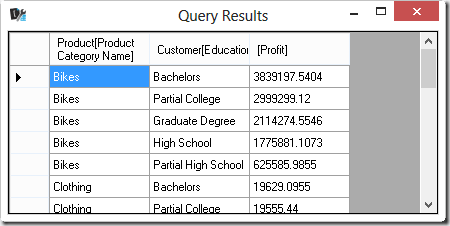In this case, applying the pattern of moving the extended columns out of a SUMMARIZE into an ADDCOLUMNS does not work, because the GENERATE used as a parameter of the SUMMARIZE returns only a few products and customers – while the SUMMARIZE only considers the sales related to these combinations of products and customers. Consider the following query and its result – please note that the GENERATE statement is included within a CALCULATETABLE statement, so that it transforms the row context of the ADDCOLUMNS statement into a filter context for executing the GENERATE statement only for the products of the current category:

```EVALUATE
SUMMARIZE(
GENERATE(
Product,
TOPN(
2,
Customer,
CALCULATE( SUM( 'Internet Sales'[Sales Amount] ) )
)
),
Product[Product Category Name],
Customer[Education]
),
"Profit",
CALCULATE(
SUM( 'Internet Sales'[Gross Profit] ),
CALCULATETABLE(
GENERATE(
Product,
TOPN(
2,
ALL( Customer[CustomerKey] ),
CALCULATE( SUM( 'Internet Sales'[Sales Amount] ) )
)
)
)
)
)
ORDER BY [Profit] DESC
```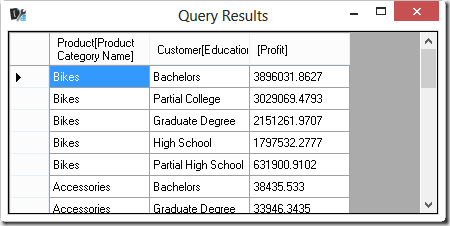As you can see, the results are different as Profit is higher than the initial result. This is because this query is considering the top 2 customers for each customer Education for each product within the same category – whereas the original query was considering the top 2 customers for each product and in case these 2 customers had different Education, only a single customer for a certain product would be contributing to the result of the query.

If you wrap the SUMMARIZE into an ADDCOLUMNS, the extended columns created in ADDCOLUMNS work on a filter context defined by Product Category and Customer Education, considering many more sales than those originally used by the initial query. Thus, in order to generate the equivalent result by using ADDCOLUMNS, it is necessary to replicate the GENERATE operation in a CALCULATETABLE statement – but because we need to include the Product Category and Customer Education calculation into the output, we also need to alter the original GENERATE in order to remove the part of the filter context that might alter the calculation used by TOPN.

This is the equivalent DAX query using ADDCOLUMNS for generating the extended column:

```EVALUATE
SUMMARIZE(
GENERATE(
Product,
TOPN(
2,
Customer,
CALCULATE( SUM( 'Internet Sales'[Sales Amount] ) )
)
),
Product[Product Category Name],
Customer[Education]
),
"Profit",
CALCULATE(
SUM( 'Internet Sales'[Gross Profit] ),
CALCULATETABLE(
GENERATE(
Product,
TOPN(
2,
ALL( Customer[CustomerKey] ),
CALCULATE(
SUM( 'Internet Sales'[Sales Amount] ),
ALL( Customer[Education] )
)
)
)
)
)
)
ORDER BY [Profit] DESC
```You should observe that the inner GENERATE uses the single column Customer[CustomerKey] instead of the Customer table, because it is necessary to interact with the external filter context to produce the accurate result. The explanation of all the details of this query could be longer, but it is out of the scope of this article. The conclusion is that extended columns in a SUMMARIZE expression should not be moved out to an ADDCOLUMNS if the table used in SUMMARIZE has particular filters and the extended column expression uses columns that are not part of the output. Even though you can create an equivalent ADDCOLUMNS query, the result is much more complex and there are no performance benefits in this refactoring. The more complex query has the exact same (not so good) performance as the SUMMARIZE query – both queries in this section require almost 20 seconds to run on Adventure Works 2012 Tabular.